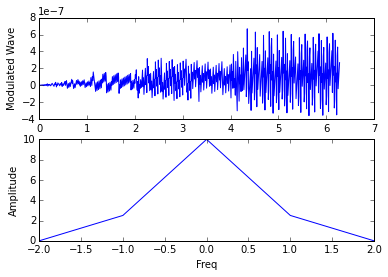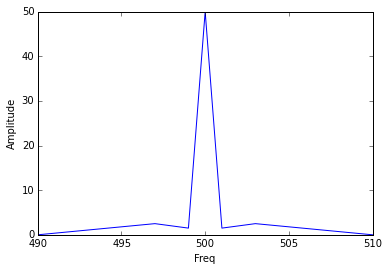# Chapter No. 3 - Modulation¶

## Example3.1, page no 135¶

In :
from __future__ import division
from math import sqrt
#Given
Ic=10 #carrier current in Amps
Imod=11.6# Current after modulation
Pmod=Rl*Imod**2#power before modulation
Ma= sqrt(2*((Pmod/Ic**2)-1))#percentage modulation
Pc=10
Pmod=Pc*(1+(Ma**2/2))#power after modulation
print 'percentage modulation is:%0.2f.2f \n Power after modulation is:%0.2f.2f watts'%(Ma*100,Pmod)

percentage modulation is:83.14.2f
Power after modulation is:13.46.2f watts


## Example3.2, page no 135¶

In :
from __future__ import division
from math import sqrt
#Given
Pc=9e3# Tx Power without modulation
Pmod=10.125e3#Tx Power after modulation
Ma= sqrt(2*((Pmod/Pc)-1))#depth of (percentage) modulation
print 'Depth of modulation is:%0.2f.2f'%(Ma)

Depth of modulation is:0.50.2f


## Example3.3, page no 136¶

In :
#Given
M1=0.2#depth of modulation for first tone
M2=0.4#depth of modulation for second tone
Pc=1200#Tx Power
Pmod=Pc*(1+M1**2/2+M2**2/2)#total power radiated after modulation by both the tones
print 'The total power radiated is %d watts'%(Pmod)

The total power radiated is 1320 watts


## Example3.4, page no 138¶

In :
#Given
Ebb=2e3#DC plate supply
Ecc=-500#DC grid bias
Ib=67e-3#DC plate current
Ic=30e-3#DC grid current
Egm=750#RF peak grid voltage
Pout=75#RF Power output
Ma=0.75#Depth of modulation
Paf=(Ma**2*Ebb*Ib)/(2*1)#modulating power required from the audio source
Pdc=Ebb*Ib#Power supplied by DC source
Zm=Ebb**2/Pdc#Modulator Impedance

Pd=Pdc+Paf-Pout#Plate dissipation
print 'modulating power required from the audio source\n is:%0.2f watts\n Modulator Impedance is:%0.2f ohm\n Plate dissipation is:%0.2f watts'%(Paf,Zm,Pd)

modulating power required from the audio source
is:37.69 watts
Modulator Impedance is:29850.75 ohm
Plate dissipation is:96.69 watts


## Example3.5b, page no 139¶

In :
from __future__ import division
from math import sqrt
#Given
Pd=944#Anode dissipation of the class C amplifier in watts
Ma=0.6#modulation depth,
Etta=0.6#efficiency
Pout=(Etta*Pd/(1-Etta))#power dissipation at 60% modulation
Pc=Pout/(1+(Ma**2/2))#Tx power
Psb=Pout-Pc
Pdc1=Pc/Etta#DC power inputto PA
Paf=Psb/Etta# modulation power input to PA
Eff=0.25# efficiency of the modulator
Pdc2=Paf/Eff#DC power input to modulator
Pdct=Pdc1+Pdc2#Total DC power to the system
Effo=Pout/Pdct#Overall Efficiency
Ma=1# 100% modulation
Pt=Pc*(1+(Ma**2)/2)
Psb=(Pc*Ma**2)/2
Paf=Psb/Etta#modulating input power to PA
Pdc2=Paf/Eff# DC power input to modulator
Pd=Pdc2-Paf#Power dissipation at the modulator
Effo1=Pout/(Pdc1+Pdc2)#Overall Efficiency
print 'Tx power:%0.2f Watts\n Power dissipation at the modulator is: %0.2f Watts\n Overall Efficiency at0.6 modulation is:%0.2f%c '%(Pc,Pd,100*Effo,'%')

Tx power:1200.00 Watts
Power dissipation at the modulator is: 3000.00 Watts
Overall Efficiency at0.6 modulation is:41.16%


## Example3.6, page no 141¶

In :
from __future__ import division
from math import sqrt#Given
Pdc=1400#DC power i/p to PA under 100% modulation
Ptdc=400#Plate dissipation
Pd=Ptdc*(2/3)#DC plate dissipation

Pdmod=Ptdc*(1/3)#
Pc=Pdc-Pd#Carrier Power

Psb=Pc/2#side band power
Paf=Psb+Pdmod#output power of modulator

Mod_Eff=0.6
Pdc2=Paf/Mod_Eff#DC i/p power to the modulator
Pd_AF=Pdc2-Paf#Plate dissipation inthe modulator
print 'Carrier Power is: %0.2f watts \n DC plate dissipation is: %0.2f watts\n output power of modulator is: %0.2f watts\n Plate dissipation inthe modulator is:%0.2f watts'%(Pc,Pd,Paf,Pd_AF)

Carrier Power is: 1133.33 watts
DC plate dissipation is: 266.67 watts
output power of modulator is: 700.00 watts
Plate dissipation inthe modulator is:466.67 watts


## Example3.7, page no 141¶

In :
#Given
Paf=500#Modulator output power
Eff=0.75#Efficiency of the amplifier
P_lost=Paf*(1-Eff)#modulating power lost in the amplifier
Psb=Paf*Eff#side band power

m=1
Pc=2*Psb

Pt=Pc+Psb#Total RF power
print 'Maximum carrier power is: %d watts\n Total RF power is: %d watts'%(Pc,Pt)

Maximum carrier power is: 750 watts
Total RF power is: 1125 watts


## Example3.8, page no 143¶

In :
from __future__ import division
from math import sqrt#Given
Po=3000# Rating of Power Amplifier
Pr=750#Push-Pull amplifier rated as
Paf=2*Pr#Rated power output from Push-Pull modulator
Eff=0.6
P_lost=Paf-(Eff*Paf)#Modulation power lost
Psb=Paf-P_lost#side band power

Pc=Po-Psb#Carrier power
Ma=sqrt(2*Psb/Pc)*100#Maximum depth of modulation
print 'Carrier power is: %d watts\n Maximum depth of modulation is: %0.2f'%(Pc,Ma)

Carrier power is: 2100 watts
Maximum depth of modulation is: 92.58


## Example3.9, page 143¶

In :
from numpy import arange, pi
#Given
t=arange(0,10,0.001)
#e=500*(1+(0.4*sin(3140*t)))*sin(6.28e7*t)
#a
wc=6.28e7#Carrier angular frequency
fc=wc/(2*pi)# Carrier freq
#b
wm=3140#Modulating angular freq
fm=wm/(2*pi)#Modulating freq
#c
Ec=500#/peak carrier voltage
Pc=(Ec**2)/(2*600)#Carrier power
#d
Ma=0.4
Pt=Pc*(1+(Ma**2 / 2))#Mean output power
#e
Ecp=Ec+(Ma*Ec)#Peak output voltage
Ptm=Ecp**2/(2*Rl)#Peak power
print 'Carrier freq is: %d MHz\nModulating freq is:%d Hz\nCarrier power is: %0.2f watts\nMean output power is: %0.2f watts\nPeak output power is: %0.2f watts'%(round(fc*1e-6),round(fm),Pc,Pt,Ptm)

Carrier freq is: 10 MHz
Modulating freq is:500 Hz
Carrier power is: 208.33 watts
Mean output power is: 225.00 watts
Peak output power is: 408.33 watts


## Example3.10, page no 143¶

In :
from math import atan,sqrt,pi
from cmath import polar
#Given
#b
Pc=50e3#Carrier power
Z=36 + 1J*40#base impedance of the antenna
Ma=1#modulation depth
Pmod=Pc*(1+((Ma**2)/2))#power delivered to the antenna under 100% modulation
#i
R=36#resistance of the antenna
Irms=sqrt(Pmod/R)#Antenna Current

#ii
Ic=sqrt(Pc/R)#RMS carrier current

Icm=Ic*sqrt(2)# Peak carrier current
Imod=2*Icm#Modulated current

Theta=atan(40/36)*180/pi# from real and imaginary components of Z
Vbm100=Imod*Z#Peak base output voltage for 100% modulation
[Re_Vb,Im_Vb]=polar(Vbm100)

#iii
Ma=0.5
Imod=Icm*(1+0.5)

Vbm50=Imod*Z
[Re_Vb1,Im_Vb1]=polar(Vbm50)
print 'Antenna current for full modulation is: %0.2f amp\nPeak base voltage is: %0.2f/_%d volts\nPeak base voltage is: %0.2f/_%d volts'%(Irms,Re_Vb,Theta,Re_Vb1,Theta)
# The Ans is little deviated from that of book as the decimal places considered while calculating at different stages might be different

#Answers from the book are little deviated but the evaluated values in the scilab are correct results

Antenna current for full modulation is: 45.64 amp
Peak base voltage is: 5672.55/_48 volts
Peak base voltage is: 4254.41/_48 volts


## Example3.11, page no 144¶

In :
from math import sin,pi
from numpy import arange
%matplotlib inline
from matplotlib.pyplot import plot, subplot, title, xlabel, ylabel, show
# Given
def f(x):
y=[]
for xx in x:
y.append(Ec*(1+ma*(sin(wm*xx)))*sin(wc*xx))
return y
Ec=10
ma=0.5
wm=10000*pi
wc=2*pi*1e7
x=arange(0,20*pi/10,.01)
subplot(2,1,1)
plot(x,f(x))
xlabel("t")
ylabel("Modulated Wave")
Fc=wc/(2*pi)
Fm=wm/(2*pi)
Fusb=(wm+wc)/(2*pi)
Flsb=(wm-wc)/(2*pi)
print 'USB freq=%d k5Hz\nUSB amplitude=%0.2f V\nLSB freq=%d kHz\nLSB amplitude=%0.2f V\nCarrier amplitude=%d V'%(Fusb*1e-3,2.5,Flsb*-1e-3,2.5,10)
F=[0,2.5,10,2.5,0]
T=[-2,-1,0,1,2]
subplot(2,1,2)
plot(T,F)
xlabel("Freq")
ylabel("Amplitude")
show()

USB freq=10005 k5Hz
USB amplitude=2.50 V
LSB freq=9995 kHz
LSB amplitude=2.50 V
Carrier amplitude=10 V## Example3.12 page no 145¶

In :
from math import sqrt
#Given
Pc=9e3#unmodulated carrier power
Pt=10.125e3#Modulated carrier power
Ma=sqrt(2*((Pt/Pc)-1))#depth of modulation
print 'The depth of modulation is: %d%c'%(Ma*100,'%')

The depth of modulation is: 50%


## Example3.13 page no 148¶

In :
#Given
Pt=5e3#carrier power for 95% modulation
Ma=0.95
Pc=Pt/(1+((Ma**2)/2))#carrier power
Ma=0.2#average modulation by speech signal
Psb=(Ma**2)*Pc/2#the power n the sideband
Pout=Psb/2# because one of the side band is suppressed
print 'The power output is: %0.2f W'%(Pout)

The power output is: 34.45 W


## Example3.14 page no 152¶

In :
#Given
#Phi=(wc*t+Mf*sin(wmt))....instantaneous phase of FM
fm=5000#modulating freq
deltaf=50e3#freq deviation
deltaPhi1=deltaf/fm# Advance or retard in phase

fm=100#modulating freq in second signal
deltaPhi2=deltaf/fm

DeltaPhi1= 10 rad



## Example3.14 page no 157¶

In :
from __future__ import division
from math import sqrt,atan
#Given
#e=Ec(1+0.4cos(2pie3*t))*sin(2pie7*t)
fm=1000#modulating s/g freq
deltaTheta=2*atan(0.4)#peak phase deviation

deltaF=deltaTheta*fm#Peak freq deviation

Ec=1
Er=sqrt((Ec**2)*(1+(0.4**2)))
m=(Er-Ec)/Ec#depth of residual AM

AMFr=2*fm# freq ofresidual AM
print 'Peak Phase Deviation: %0.2f rad\nPeak Freq Deviation: %d Hz\nDepth of residual AM: %0.2f\nResidual AM freq:%d kHz'%(deltaTheta,deltaF,(round(m*100)/100),AMFr*1e-3)

Peak Phase Deviation: 0.76 rad
Peak Freq Deviation: 761 Hz
Depth of residual AM: 0.08
Residual AM freq:2 kHz


## Example3.16 page no 170¶

In :
#Given
deltaF=25e3#freq deviation
#a
fm=100#modulation signal freq
mf=deltaF/fm# Max phase deviation
print 'a)'
print 'Max phase deviation is:%d rad'%(mf)
#b
fm=10e3#modulation signal freq
mf=deltaF/fm#Max phase deviation

print 'b)'
print 'Max phase deviation is:%0.2f rad'%(mf)

a)
b)


## Example3.17, page no 171¶

In :
from __future__ import division
from math import sqrt
#Given
gm=0.1e-3# trans-conductance variation A/V
C=0.5e-12# capactance between anode and grid
R=1e3# resistance
fo=10e6# oscillator freq
Vrms=1.414#AF RMS voltage
Vp=sqrt(2)*Vrms#Peak voltage
Ct=100e-12#tank capacitance
deltaC=gm*C*R*Vp

deltaF=fo*(deltaC/(2*Ct))# maximum freq deviation
print 'The maximum freq deviation is: %d kHz'%(round(deltaF/1000))

The maximum freq deviation is: 5 kHz


## Example3.18, page no 172¶

In :
#Given
deltaF=1e6# max freq deviation
fm=10e3#modulating freq
mf=(2*deltaF)/fm# modulation coefficient
BW=mf*fm# bandwidth
print 'The approximate bandwidth is: %d MHz'%(BW/1e6)

The approximate bandwidth is: 2 MHz


## Example3.19, page no 172¶

In :
#Given
deltaF=75e3# max freq deviation
fm=15e3#modulation freq
mf=(2*deltaF)/fm# freq modulation depth
BW=mf*fm# Bandwidth
print 'The approximate bandwidth is: %d kHz'%(BW/1e3)

The approximate bandwidth is: 150 kHz


## Example3.21, page no 173¶

In :
#Given
deltaF=75e3#freq deviation
fm=15e3# modulating freq
mf=deltaF/fm
BW=2*mf*fm# Bandwidth
GB=25e3#Guard Band
BWo=BW+(2*GB)# Overall bandwidth
print 'Overall bandwidth including guard band is %d kHz'%(BWo/1e3)

Overall bandwidth including guard band is 200 kHz


## Example3.25, pageno 175¶

In :
from math import sin
%matplotlib inline
from matplotlib.pyplot import plot, subplot, title, xlabel, ylabel, show
#Given
#em=3sin(2*pi*1000t)+5cos(2*pi*3000t)
#ec=50sin(2*pi*500e3*t)
m1=0.06#(sine wave amplitude/ peak carrier voltage)
m2=0.1#(cosine wave amplitude/ peak carrier voltage)
Vc=50#Carrier voltage
Pc=(Vc**2)/(2*R)#Peak carrier power
Pt=Pc*(1+((m1**2+m2**2)/2))#Total power after modulation
print 'Average power is: %0.2f watts'%(Pt)
F=[0,2.5,1.5,50,1.5,2.5,0]
T=[490,497,499,500,501,503,510]
plot(T,F)
xlabel("Freq")
ylabel("Amplitude")
show()

Average power is: 25.17 watts## Example3.26, page no 176¶

In :
#Given
mp=0.1#Modulating index
fm=400#Modulating signal freq
deltaF=mp*fm#Max freq deviation
#print deltaF)
ReqDev=50e3# Required deviation
MF=ReqDev/deltaF# multiplication factor
print 'Required Deviation is: %d kHz\n'%(ReqDev/1e3)
print 'Required Multipication Factor is: 5*5*5*5*2'

Required Deviation is: 50 kHz

Required Multipication Factor is: 5*5*5*5*2


## Example3.27, page no 176¶

In :
#Given
Q=100 #Q factor
fc=1000e3# Carrier freq
fsb1=999e3#lower Side band freq
fsb2=1001e3#Upper side Band freq
ma=0.5#Modulation depth of signal current
Ma=ma/1.019# Expression for Ma after simplification
print 'The Depth of modulation across the \n circuit is : Ma= %0.2f%c'%(Ma*100,'%')

# Note :  There are some calculation errors in the solution presented in the book

The Depth of modulation across the
circuit is : Ma= 49.07%


## Example3.28, page no 177¶

In :
from math import sqrt
#Given
R=1#Antenna Resistance assumed to be 1 ohm for ease of calculation
Ic=10.8# current with no modulation
Pc=Ic**2*R#power with no modulation
It=12.15#modulated current
Pt=It**2*R# modulated power
ma=(sqrt(2*(((It/Ic)**2)-1)))#modulation depth)

print 'Depth of modulation:%0.2f %c'%(round(1000*ma)/10,'%')#

Depth of modulation:72.90 %


## Example3.29, page no 177¶

In :
#Given
Pc=100e3#Carrier power
ma=0.5#Depth of modulation
Pt=Pc*(1+((ma**2)/2))#total RF power
print 'Total RF power delivered is:Pt= %0.2f kW'%(Pt/1e3)

Total RF power delivered is:Pt= 112.50 kW


## Example3.30, page no 178¶

In :
#Given
Pt=100e3# Total power
ma=0.9#Depth of modulation
Pc=Pt/(1+((ma**2)/2))#Carrier power
Psb=Pt-Pc# Intelligence power i.e sideband power
print 'Carrier power:%0.2f kW\nThe Intelligence power: %0.2f kW'%(Pc/1000,Psb/1000)

Carrier power:71.17 kW
The Intelligence power: 28.83 kW


## Example3.19, page no 178¶

In :
from math import sin,sqrt
#Given

Modulation Index is: 64.81